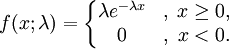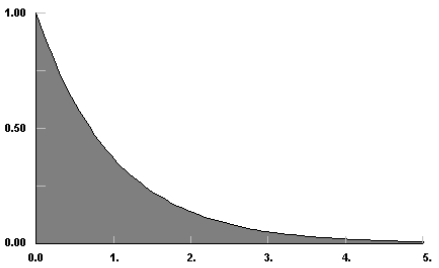AnyLogic

# exponentialThe Exponential distribution is a continuous distribution bounded on the lower side. Its shape is always the same, starting at a finite value at the minimum and continuously decreasing at larger x. The Exponential distribution decreases rapidly for increasing x.

The Exponential distribution is frequently used to represent the time between random occurrences, such as the time between arrivals at a specific location in a queuing model or the time between failures in reliability models. It has also been used to represent the services times of a specific operation. Further, it serves as an explicit manner in which the time dependence on noise may be treated. As such, these models are making explicit use of the lack of history dependence of the exponential distribution; it has the same set of probabilities when shifted in time. Even when Exponential models are known to be inadequate to describe the situation, their mathematical tractability provides a good starting point. Later, a more complex distribution such as Erlang or Weibull may be investigated.

#### Example

lambda = 1; min = 0### exponential(double lambda, double min)

Description
Generates a sample of the Exponential distribution.
Parameters
Name Type of value Description
lambda double The shape parameter.
min double The minimum x value.
Result
Type Description
double The generated sample.

### exponential(double lambda)

Description
Generates a sample of the Exponential distribution with min set to 0. Is equivalent to exponential(lambda, 0).
Parameters
Name Type of value Description
lambda double The shape parameter.
Result
Type Description
double The generated sample.

### exponential()

Description
Generates a sample of the Exponential distribution with lambda set to 1 and min set to 0. Is equivalent to exponential(1, 0).
Result
Type Description
double The generated sample.

### exponential(double lambda, double min, java.util.Random r)

Description
Generates a sample of the Exponential distribution using the specified random number generator.
Parameters
Name Type of value Description
lambda double The shape parameter.
min double The minimum x value.
r java.util.Random The random number generator.
Result
Type Description
double The generated sample.

This document includes content from the “Stat::Fit User’s Manual”. Copyright 2016 Geer Mountain Software Corp.